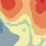624
4
11-27-2018 02:34 AMNew Contributor II

Hi! I have a script with a set of environment properties. I'm having a problem to save my outputs due to Error 999998. After some attempts i have figured out that the problem occurs when I set a mask for the environment (a shapefile). If I remove the mask, then my outputs are saved.

Any idea how can I solve this issue?

The error (RuntimeError: Error 999998: Unexpected Error) happens in line 60 of the following script:

``````import os
import arcpy
from arcpy.sa import *

# Checking spatial analyst extension
if arcpy.CheckExtension('Spatial') == 'Available':
arcpy.CheckOutExtension('Spatial')
else:
print('Spatial Analyst extension is not available')

# Setting paths
inp_ws = os.path.join("D:\\", "master", "data", "cb")
out_ws = os.path.join(inp_ws, "results")
temp_ws = os.path.join(inp_ws, "temp")
lu_folder = os.path.join(inp_ws, "lu")

# Setting geoprocessing environments
arcpy.env.workspace = inp_ws
arcpy.env.scratchWorkspace = temp_ws
arcpy.env.overwriteOutput=True
arcpy.env.rasterStatistics = "STATISTICS 1 1"
arcpy. env.snapRaster = "soc_default"
arcpy.env.cartographicCoordinateSystem = "PROJCS['South_America_Albers_Equal_Area_Conic',GEOGCS['GCS_South_American_1969',DATUM['D_South_American_1969',SPHEROID['GRS_1967_Truncated',6378160.0,298.25]],PRIMEM['Greenwich',0.0],UNIT['Degree',0.0174532925199433]],PROJECTION['Albers'],PARAMETER['False_Easting',0.0],PARAMETER['False_Northing',0.0],PARAMETER['Central_Meridian',-60.0],PARAMETER['Standard_Parallel_1',-5.0],PARAMETER['Standard_Parallel_2',-42.0],PARAMETER['Latitude_Of_Origin',-32.0],UNIT['Meter',1.0]]"

# Describing rasters file extension
for dirpath, dirnames, filenames in arcpy.da.Walk(inp_ws, datatype="RasterDataset"):
dir_lu = [d for d in dirnames if d == 'lu']
dirnames = dir_lu
if dirpath == inp_ws or dirpath == lu_folder:
print"\nThe formats of input rasters in folder {} are:".format(dirpath)
for f in filenames:
path = os.path.join(dirpath, f)
ext = arcpy.Describe(path).format
print  "< {0} > for {1}".format(ext, f)

# Cataloging LU maps in a list
lu_scenarios = []
for dirpath, dirnames, filenames in arcpy.da.Walk(lu_folder, datatype='RasterDataset'):
for f in filenames:
lu_scenarios.append(os.path.join(dirpath,f))

# Setting input data
climate = Raster("climate")
soc_default = Raster("soc_default")
codes_soc = "soc_random_test.txt"
codes_bc = "bc_stock.txt"

for (i, lu_scenario) in enumerate(lu_scenarios):
lu = Raster(lu_scenario)
# map algebra: combine climate + lu files
clim_lu = climate + lu
# reclass by soc factor
soc_factor = ReclassByTable(clim_lu, codes_soc, "Code", "Code", "Value")
# map algebra: create soc stock
soc = (soc_factor*soc_default)/1000 #tonne C/ha
# reclass by bc stock
bc = ReclassByTable(clim_lu, codes_bc, "Code", "Code", "Value") # map algebra: soc stock + bc stock
tc = soc + bc
if str(tc) != "":
tc_output = (temp_ws + "\\" + str(i)+"-tc-test")
tc.save(tc_output)
print tc‍‍‍‍‍‍‍‍‍‍‍‍‍‍‍‍‍‍‍‍‍‍‍‍‍‍‍‍‍‍‍‍‍‍‍‍‍‍‍‍‍‍‍‍‍‍‍‍‍‍‍‍‍‍‍‍‍‍‍‍‍‍‍‍‍‍‍‍‍‍‍‍‍‍‍‍‍‍‍‍‍‍‍‍‍‍‍‍‍‍‍‍‍‍‍‍‍‍‍‍‍‍‍‍‍‍‍‍‍‍‍‍‍‍‍‍‍‍‍‍‍‍‍‍‍‍``````

Thank you very much!!

Tags (1)
1 Solution

Accepted SolutionsbyMVP Esteemed Contributor

line 22 above, there is a space that shouldn't be there is that a copy error?  What is the actual folder name for your workspace?  If you are using a shapefile you are missing the *.shp if the file is in a folder

4 RepliesbyMVP Esteemed Contributor

line 22 above, there is a space that shouldn't be there is that a copy error?  What is the actual folder name for your workspace?  If you are using a shapefile you are missing the *.shp if the file is in a folderNew Contributor II

Line 22 was a copy error. My worskpace in inp_ws but i'm saving in temp_ws, And yeah, the problem was the missing *.shp! My bad. My bad, thanks. Now is saving, but it takes 9x more time to finish... If someone has a wiser idea to reduce it, I would appreciateThanks again!Regular Contributorby`` if str(tc) != "":‍``